# PSAT Math : How to find the length of the side of a right triangle

## Example Questions

2 Next →

### Example Question #461 : Psat Mathematics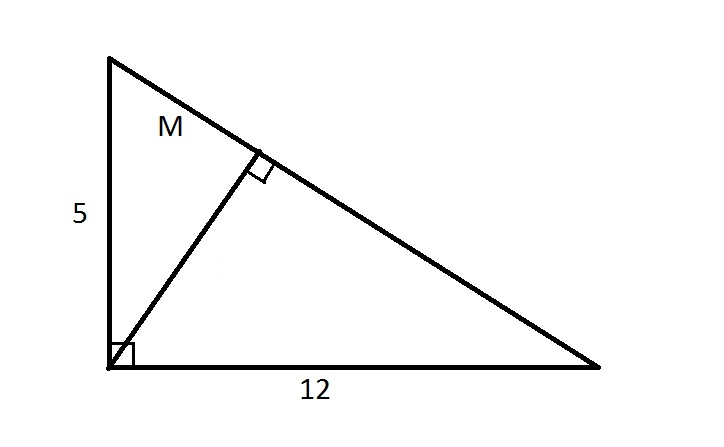Note: Figure NOT drawn to scale.

Refer to the above diagram. Evaluate.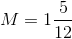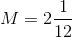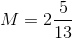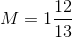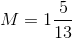Explanation:

The altitude perpendicular to the hypotenuse of a right triangle divides that triangle into two smaller triangles similar to each other and the large triangle. Therefore, the sides are in proportion. The hypotenuse of the triangle is equal to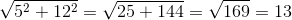Therefore, we can set up, and solve forin, a proportion statement involving the shorter side and hypotenuse of the large triangle and the larger of the two smaller triangles: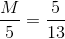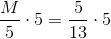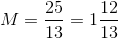2 Next →

### All PSAT Math Resources